## Algebra

Showing posts with label quadratic form. Show all posts
Showing posts with label quadratic form. Show all posts

### Solving Equations Quadratic in Form

In this section, we make use of all the techniques that we have learned so far for solving quadratic equations. In fact, the equations found here are reducible to quadratic form.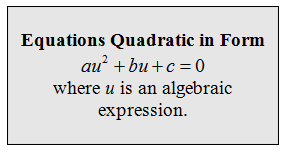Here are most of the reducible equations that we are likely to encounter. Begin by trying to identify what can be squared to obtain the leading variable term.Tip: Look at the middle term for a hint as to what u should be.In the previous solved problem, we certainly could have distributed the expression on the left side, put the equation in standard form then re-factored it. Instead, here we are illustrating a technique that will be used to easily solve many other equations that are quadratic in form.

Solve by making a u-substitution.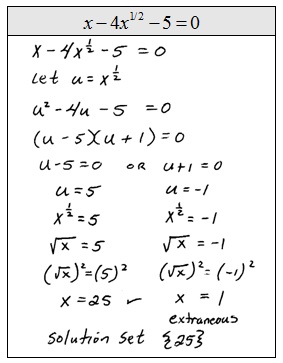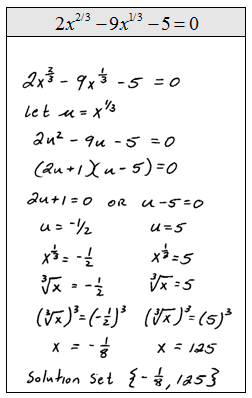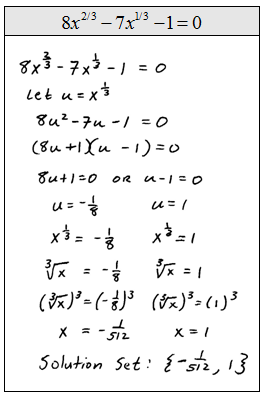Solve: x^6 + 26x^3 -27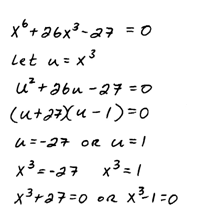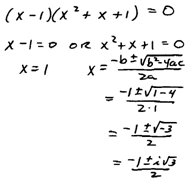Six Answers: { -3, 1, (3±3iSqrt(3))/2, (-1±iSqrt(3))/2 }

So far we have been able to factor after we make the u-substitution.  If the resulting quadratic equation does not factor, then use the quadratic formula.